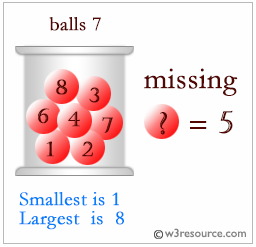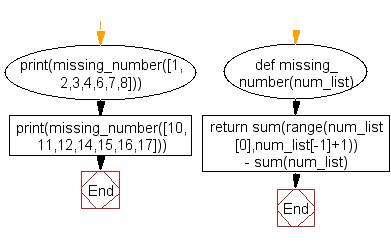﻿ Python: Find a missing number from a list - w3resource# Python Challenges: Find a missing number from a list

## Python Challenges - 1: Exercise-7 with Solution

Write a Python program to find a missing number from a list.

Explanation:Sample Solution:-

Python Code:

``````def missing_number(num_list):
return sum(range(num_list,num_list[-1]+1)) - sum(num_list)

print(missing_number([1,2,3,4,6,7,8]))

print(missing_number([10,11,12,14,15,16,17]))
```
```

Sample Output:

```5
13
```

Flowchart:## Visualize Python code execution:

The following tool visualize what the computer is doing step-by-step as it executes the said program:

Python Code Editor:

What is the difficulty level of this exercise?

Test your Python skills with w3resource's quiz

﻿

## Python: Tips of the Day

Python: Kwargs

**kwargs and *args are function arguments that can be very useful.

They are quite underused and often under-understood as well.

Let's try to explain what kwargs are and how to use them.

• While *args are used to pass arguments at an unknown amount to functions, **kwargs are used to do the same but with named arguments.
• So, if *args is a list being passed as an argument, you can think of **kwargs as a dictionary that's being passed as an argument to a function.
• You can use arguments as you wish as long as you follow the correct order which is: arg1, arg2, *args, **kwargs. It's okay to use only one of those but you can't mix the order, for instance, you can't have: function(**kwargs, arg1), that'd be a major faux pas in Python.
• Another example: You can do function(*args,**kwargs) since it follows the correct order.
• Here is an example. Let's say satelites are given with their names and weight in tons in dictionary format. Code prints their weight as kilograms along with their names.
```def payloads(**kwargs):
for key, value in kwargs.items():
print( key+" |||", float(value)*100)
payloads(NavSat1 = '2.5', BaysatG2 = '4')
```

Output:

```NavSat1 ||| 250.0
BaysatG2 ||| 400.0```

Since the function above would work for any number of dictionary keys, **kwargs makes perfect sense rather than passing arguments with a fixed amount.

```def payloads(**kwargs):
for key, value in kwargs.items():
print( key+" |||", float(value)*100)

sats={"Tx211":"3", "V1":"0.50"}
```Tx211 ||| 300.0